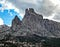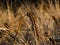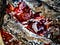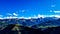# Double Deep Q-Networks

Today’s post is about Double Deep Q-Networks. Double Deep Q-Networks is an important enhancement of the standard Deep Q-Networks that I explained in my previous post. We will first see what is the problem with the DQN and how we encounter these problems using the Double DQN. After that, we will implement the Double DQN from scratch, where I will explain each step of the implementation.Main du juif mountain in Algeria. Taken by me

Let’s recall an important definitions and formulas that we need to master before going deeper.

## Bellman Optimality Equation

The goal of the agent is to find a policy that satisfies the Bellman optimality equation for each state…

# PageRank algorithm, fully explained

The PageRank algorithm or Google algorithm was introduced by Lary Page, one of the founders of Google. It was first used to rank web pages in the Google search engine. Nowadays, it is more and more used in many different fields, for example in ranking users in social media etc… What is fascinating with the PageRank algorithm is how to start from a complex problem and end up with a very simple solution. In this post, I will teach you the idea and theory behind the PageRank algorithm. You just need to have some basics in algebra and Markov Chains…

# Deep Q-Networks: from theory to implementation

In my previous post, I explained and implemented the Q-learning algorithm from scratch using ForzenLake environment provided by gym library. Please be sure to have already have some Reinforcement Learning (RL) basics and have understood the Q-learning algorithm.taken by me

Well, in the Q-learning algorithm, we compute the Q-table which contains the Q-values of any state-action pair using the Q-value iteration. Q-learning algorithm works well for finite states and actions spaces because, since we store every state-action pair, this would mean that we need huge space amount of memory to store all of them and much more iterations for the Q-table to…

# Q-Learning Algorithm: From Explanation to Implementation

In my today’s medium post, I will teach you how to implement the Q-Learning algorithm. But before that, I will first explain the idea behind Q-Learning and its limitation. Please be sure to have some Reinforcement Learning (RL) basics. Otherwise, please check my previous post about the intuition and the key math behind RL.Taken by me

Well, let’s recall some definitions and equations that we need for implementing the Q-Learning algorithm.

In RL, we have an environment that we want to learn. For doing that, we build an agent who will interact with the environment through a trial-error process. At each time…

# A gentle introduction to Reinforcement Learning

In this article, I will try to explain the idea behind Reinforcement Learning and why it is much different from classic machine learning. I will also present and explain some key math formulas which are very important to understand before going deeper in Reinforcement Learning.Djurdjura Mountains in Kabylie region — Algeria- Taken by me

# What is the difference between RL and other Machine Learning field?

As we know, Machine Learning can be split into three major domains: Supervised Learning, Unsupervised Learning, and Reinforcement Learning. In both supervised and unsupervised learning, we try to learn from available data contained in a dataset in order to build the best model which generalizes to similar data that are not used during the training…## Amrani Amine

5th year computer science and statistics engineering student at Polytech Lille, I am fascinated by machine learning and Graph Neural Networks.